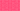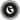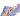PricingResources

# What is equivalent annual cost (EAC)?Written by

Deciding whether to make an investment in one asset or another can be challenging. Fortunately, there are numerous financial metrics that can assist you in the decision-making process. One of the most effective tools is the equivalent annual cost formula, which can help you understand the annual cost of an asset throughout its life. Find out everything you need to know about how to calculate equivalent annual cost with our definitive guide.

## Equivalent annual cost explained

Put simply, equivalent annual cost refers to the cost-per-year of owning, operating, and maintaining an asset over the course of its entire lifespan. Equivalent annual cost analysis is often used in the capital budgeting process, as it is an effective way to compare the cost-effectiveness of different assets. You can also use equivalent annual cost analysis to determine whether it’s better to lease or purchase an asset outright, while the calculation can also help you understand the impact that maintenance costs will have on the asset, moving forward.

## How to calculate equivalent annual cost

Learning how to calculate equivalent annual cost is relatively simple, as there’s an easy equivalent annual cost formula that you can use. Essentially, you’ll need to divide the net present value (NPV) of the asset by the present value of an annuity factor:

Where:

In this formula, “r” refers to the annual interest rate, while “t” refers to the number of periods. This can get somewhat complicated, which is why the standard equivalent annual cost formula simplifies the calculation. The formula is as follows:

In this equivalent annual cost formula, “discount rate” is the return that’s necessary to make the project viable (also referred to as the cost of capital). “n” is the number of periods.

## Equivalent uniform annual cost example

To see how equivalent annual cost works in practice, let’s take a look at an example. Imagine that Company A is choosing between investing in two competing projects: Asset 1 and Asset 2. Asset 1 has an initial capital outlay of £100,000, an expected lifespan of 5 years, and annual maintenance expenses of £4,000. By contrast, Asset 2 has an initial capital outlay of £145,000, an expected lifespan of 8 years, and annual maintenance expenses of £2,500.

Assuming a 5% cost of capital, we can calculate the annuity factor like so:

Then, you can work out the equivalent uniform annual cost – remembering to add on the annual maintenance cost:

As you can see, our equivalent annual cost analysis indicates that – purely from a financial point of view – Asset 2 is a better option as its EAC is considerably less than Asset 1.

## Limitations of equivalent annual cost analysis

The main disadvantage of equivalent annual cost analysis is the fact that you’ll need to estimate the discount rate. These types of forecasts tend to be inaccurate, which can throw the entire equivalent annual cost formula off. As such, it’s always important to remember to combine equivalent annual cost analysis with other capital budgeting tools to make sure that you’re getting the most accurate data regarding the investments that you’re comparing.

## We can help

GoCardless helps you automate payment collection, cutting down on the amount of admin your team needs to deal with when chasing invoices. Find out how GoCardless can help you with ad hoc payments or recurring payments.Contact sales

Sales

Support